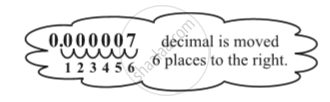Share

# Use of Exponents to Express Small Numbers in Standard Form

#### notes

Observe the following facts.
1. The distance from the Earth to the Sun is 149,600,000,000 m.
2. The average diameter of a Red Blood Cell is 0.000007 mm.
3. Thickness of Class VII Mathematics book is 20 mm.
4. Average radius of the Sun is 695000 km.
5. The height of Mount Everest is 8848 m.
Observe that there are few numbers which we can read like  8848 m, 6,95,000 km. There are some large numbers like 149,600,000,000 m and some very small numbers like 0.000007 m.
Now ,  let us try to express 0.000007 m in standard form.
0.000007 = 7 / 1000000 = 7/ 10^6 = 7 xx 10^(-6)0.000007 m = 7 xx 10^(-6) m

### Shaalaa.com

Use of Exponents to Express Small Numbers in Standard Form [00:09:23]
S
0%

S